# Math in Focus Grade 4 Chapter 3 Practice 3 Answer Key Modeling Division with Regrouping

Go through the Math in Focus Grade 4 Workbook Answer Key Chapter 3 Practice 3 Modeling Division with Regrouping to finish your assignments.

## Math in Focus Grade 4 Chapter 3 Practice 3 Answer Key Modeling Division with Regrouping

Lisa cannot remember the steps to divide.
Help her complete the steps.

Question 1.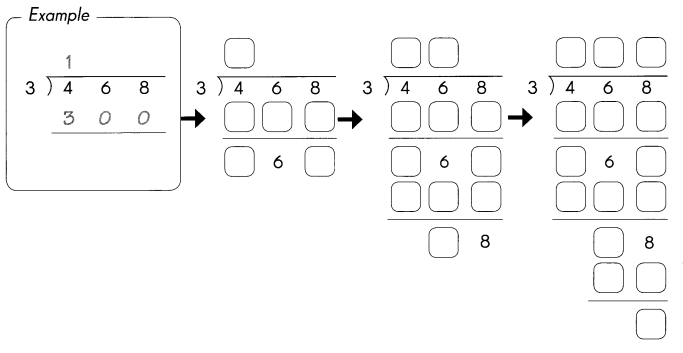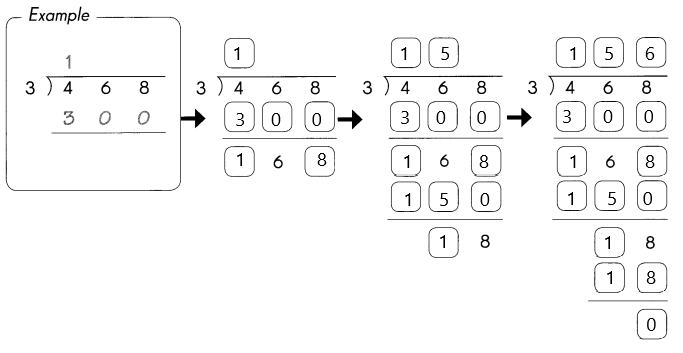Question 2.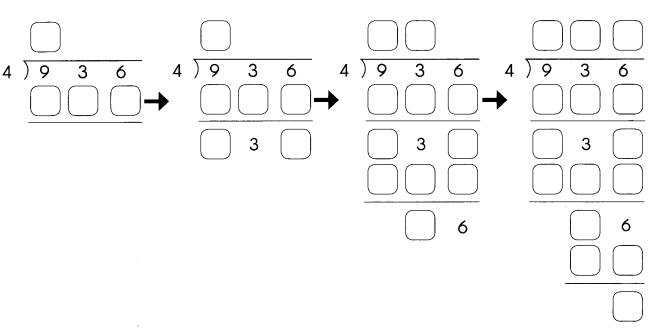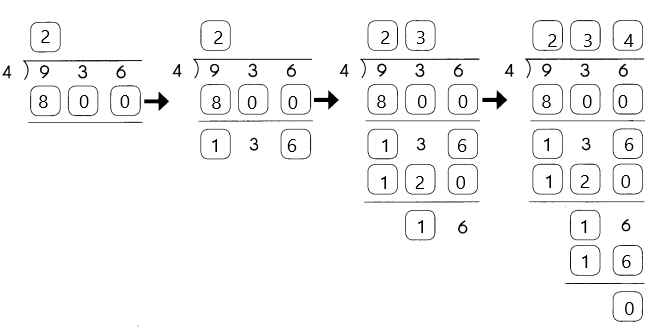Divide. Then use the quotients to complete the number puzzle.

Down

Question 3.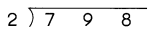798 ÷ 2 = 399.

Explanation:
The quotient of 798 ÷ 2 is 399.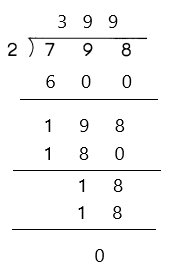Question 4.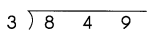849 ÷ 3 = 283.

Explanation:
The quotient of 849 ÷ 3 is 283.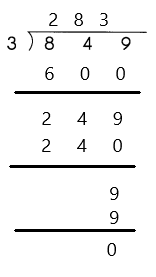Question 5.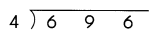696 ÷ 4 = 174.

Explanation:
The quotient of 696 ÷ 4 is 174.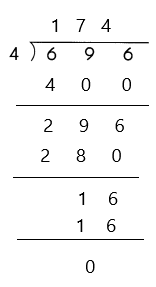Across

Question 6.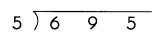695 ÷ 5 = 139.

Explanation:
The quotient of 695 ÷ 5 is 139.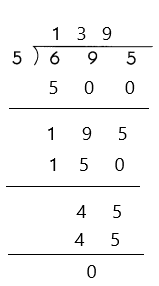Question 7.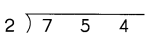754 ÷ 2 = 377.

Explanation:
The quotient of 754 ÷ 2 is 377.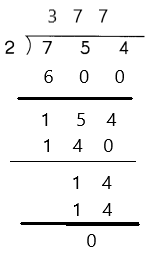Question 8.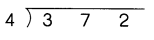372 ÷ 4 = 93.

Explanation:
The quotient of 372 ÷ 4 is 93.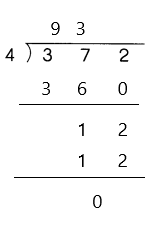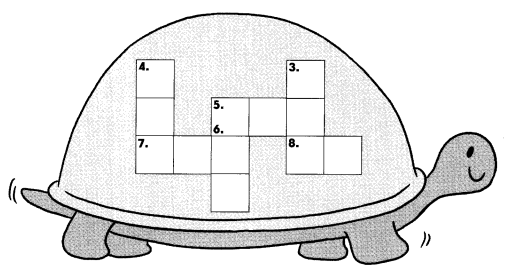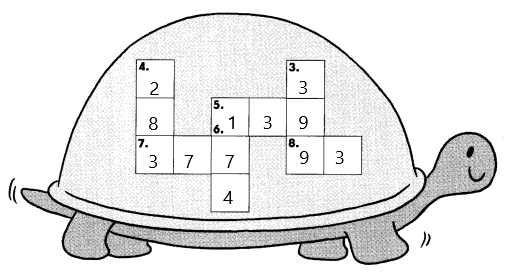Divide. Then solve the riddle.

Question 9.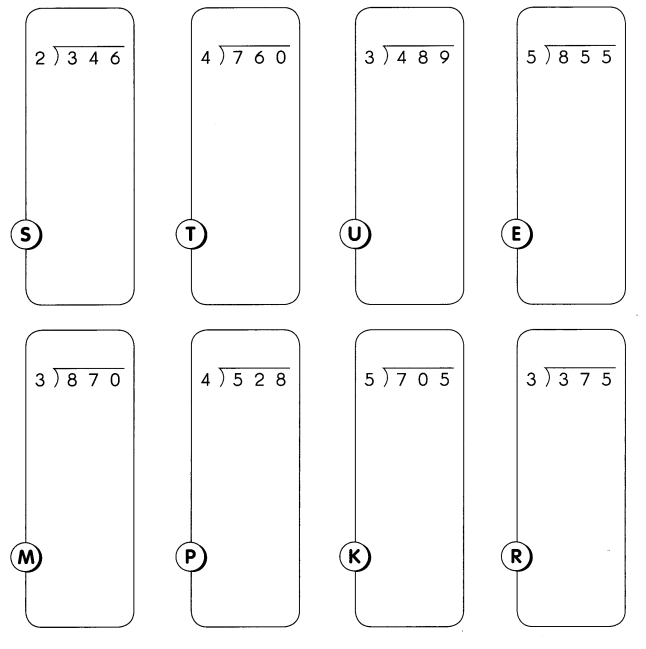Which pet makes the loudest noise?
Match the letters to the quotients below to find out.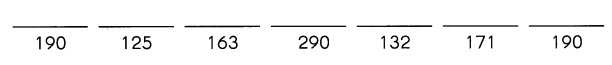The pet that makes loudest noise is Trumpet.

Explanation: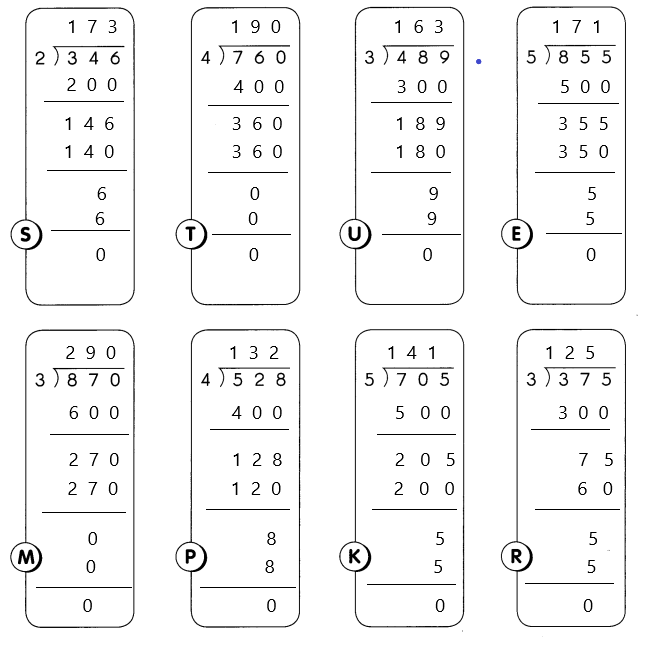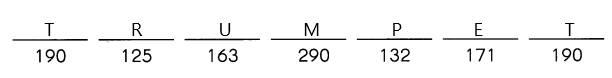Divide.

Question 10.
516 ÷ 10 = ____
516 ÷ 10 = 51 R 16.

Explanation:
By dividing 516 ÷ 10 we will get quotient as 51 with remainder 6.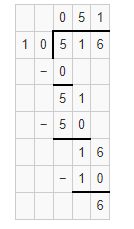Question 11.
144 ÷ 3 = ____
144 ÷ 3 = 48.

Explanation:
By dividing 144 ÷ 3 we will get quotient as 48.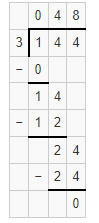Question 12.
396 ÷ 4 = ____
396 ÷ 4 = 99.

Explanation:
By dividing 396 ÷ 4 we will get quotient as 99.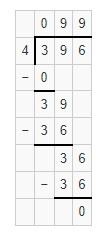Question 13.
885 ÷ 5 = ____
885 ÷ 5 = 177.

Explanation:
By dividing 885 ÷ 5 we will get quotient as 177.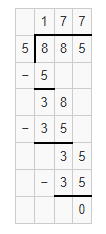Look at the steps for dividing a 3-digit number by a 1-digit number.

Example

This shows the steps in division.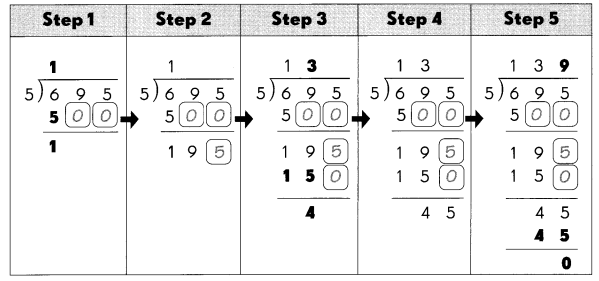Write a number for each instruction box to match the instruction with the correct step for division. The first one has been done for you.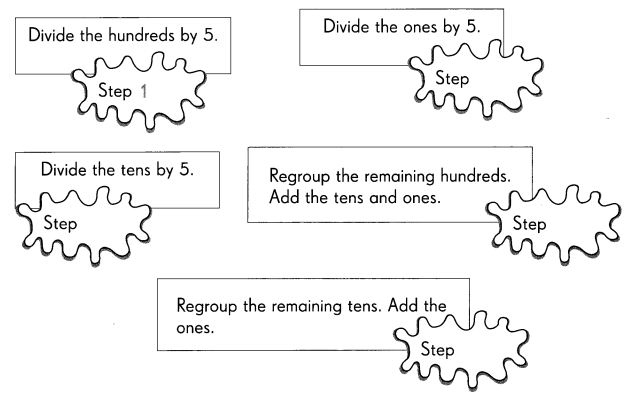Complete the division.

Question 14.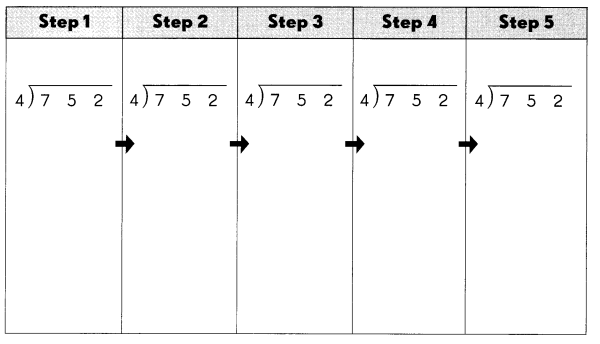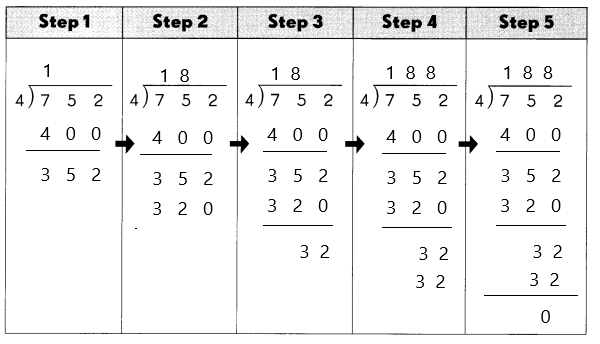Then write the steps, using the exercise on page 53 as a guide.
Step 1
___________________
Step 2
___________________
Step 3
___________________
Step 4
___________________
Step 5
___________________